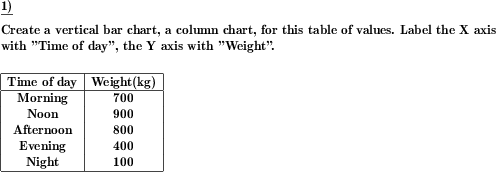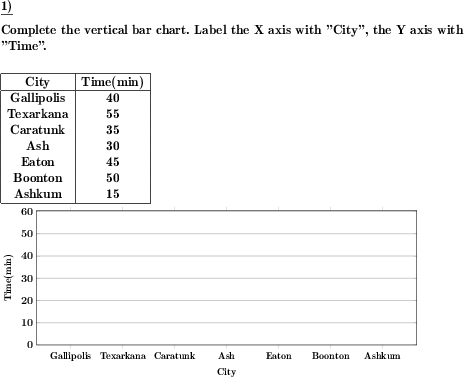Custom math worksheets at your fingertips# Details for problem "Vertical bar chart for table of values"

Quickname: 8324

Elementary School, Primary School, Junior High School, Middle School, High School.

## Summary

Create a column diagram or vertical bar chart for a given table of values.

## Examples## Description

A table of values is given. It contains one set of data assigns discrete terms such as cities, months or weekdays to variable units of measurement such as length, weight or time.

The task is to create a suitable bar chart that shows the discrete terms of the table on the X-axis and the value of the measured variable on the Y-axis.

If desired, parts of the diagram can be provided with the problem statement. So it is possible

• to provide no diagram
• to provide a diagram without columns, where only the labeling of the X or Y axes or both are specified
• to provide a diagram with axis labels where one column is already drawn in

As an additional help, a note can be included in the task definition on how to label the axes. The size of the bar chart can be selected in three steps.

Download free printable worksheets for this math problem here. The worksheet contains the problems only, the solution sheet includes the answers. Just click on the respective link.

•Worksheet 1Solution sheet with answers
•Worksheet 2Solution sheet with answers
•Worksheet 3Solution sheet with answers

If you can not see the solution sheets for download, they may be filtered out by an ad blocker that you may have installed. If this is the case, please allow ads for this page and reload the page. The solution sheets will then reappear.

• Do these sample worksheets do not really fit?
• Do you need more math worksheets, with a different level of difficulty?
• Would you like to combine different problems on a worksheet and adjust them to your needs?
• As a teacher, you can put together your own worksheets using the automatically generated math problems provided.
With a free initial credit, you can start creating your own math worksheets in a few minutes.

You can try it for free! Register here, to create custom worksheets now!

## Customization options for this problem

Parameter
Possible values
Note on axis labeling
Yes, No
Provide diagram
none, no axis labels, X axis labeled, Y axis labeled, both axes labeled, part of diagram
Size of diagram
small, medium, large

## Similar problems

Remark
Description
Same, but with a horizontal bar chart
Create a bar chart for a given table of values.
Reversed problem statement: Read values from diagram and create a table of values
Read values from a column chart or vertical bar chart and create or complete a table of values.
With multiple data sets
Create a column diagram or vertical bar chart for a given table of values with multiple data sets.